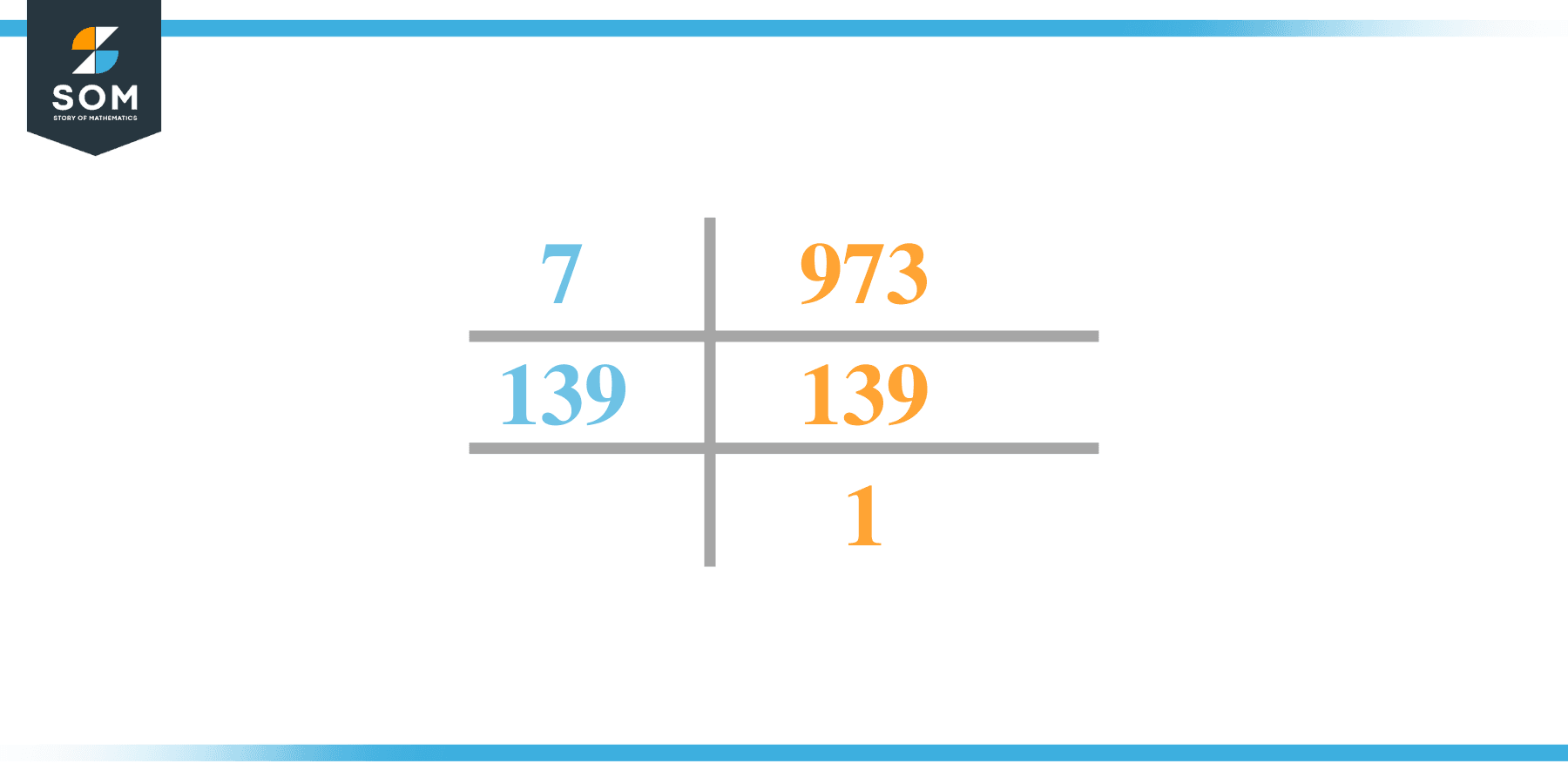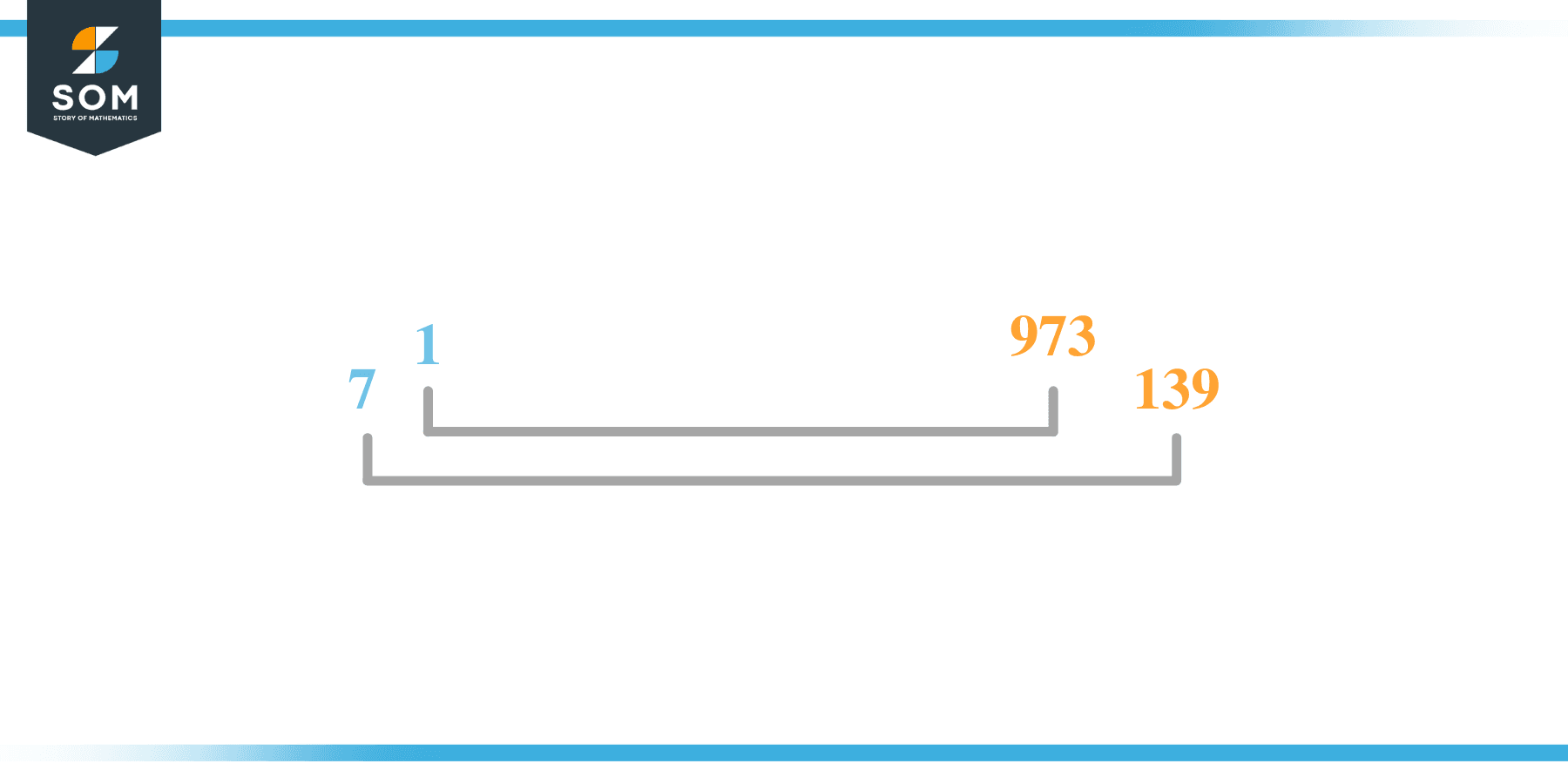# Factors of 973: Prime Factorization, Methods, and Examples

The number that leaves no remainders when divided by 973 is said to be one of its factors. There are both excellent and negative 973 variables.### Factors of 973

Here are the factors of number 973.

Factors of 973: 1, 7, 139 and 973

### Negative Factors of 973

The negative factors of 973 are similar to their positive aspects, just with a negative sign.

Negative Factors of 973: -1, -7, -139 and -973

### Prime Factorization of 973

The prime factorization of 973 is the way of expressing its prime factors in the product form.

Prime Factorization: 71 x 1391

In this article, we will learn about the factors of 973 and how to find them using various techniques such as upside-down division, prime factorization, and factor tree.

## What Are the Factors of 973?

The factors of 973 are 1, 7, 139, and 973. These numbers are the factors as they do not leave any remainder when divided by 973.

The factors of 973 are classified as prime numbers and composite numbers. The prime factors of the number 973 can be determined using the prime factorization technique.

## How To Find the Factors of 973?

You can find the factors of 973 by using the rules of divisibility. The divisibility rule states that any number, when divided by any other natural number, is said to be divisible by the number if the quotient is the whole number and the resulting remainder is zero.

To find the factors of 973, create a list containing the numbers that are exactly divisible by 973 with zero remainders. One important thing to note is that 1 and 973 are the 973’s factors as every natural number has 1 and the number itself as its factor.

1 is also called the universal factor of every number. The factors of 973 are determined as follows:

$\dfrac{973}{1} = 973$

$\dfrac{973}{7} = 139$

$\dfrac{973}{139} = 7$

$\dfrac{973}{973} = 1$

Therefore, 1, 7, 139, and 973are the factors of 973.

### Total Number of Factors of 973

For 973, there are n positive factors and n negative ones. So in total, there are m factors of 973.

To find the total number of factors of the given number, follow the procedure mentioned below:

1. Find the factorization/prime factorization of the given number.
2. Demonstrate the prime factorization of the number in the form of exponent form.
3. Add 1 to each of the exponents of the prime factor.
4. Now, multiply the resulting exponents together. This obtained product is equivalent to the total number of factors of the given number.

By following this procedure, the total number of factors of 973 is given as:

Factorization of 973 is 1 x 71 x 1391.

The exponent of 1 is 1,  7 is 1, and 139 is 1.

Adding 1 to each and multiplying them together results in 8.

Therefore, the total number of factors 973 is 8. 4 are positive, and 4 factors are negative.

### Important Notes

Here are some essential points that must be considered while finding the factors of any given number:

• The factor of any given number must be a whole number.
• The factors of the number cannot be in the form of decimals or fractions.
• Factors can be positive as well as negative.
• Negative factors are the additive inverse of the positive factors of a given number.
• The factor of a number cannot be greater than that number.
• Every even number has 2 as its prime factor, the smallest prime factor.

## Factors of 973 by Prime FactorizationThe number 973 is a composite number. Prime factorization is a valuable technique for finding the number’s prime factors and expressing the number as the product of its prime factors.

Before finding the factors of 973 using prime factorization, let us find out what prime factors are. Prime factors are the factors of any given number that are only divisible by 1 and themselves.

To start the prime factorization of 973, start dividing by its most minor prime factor. First, determine that the given number is either even or odd. If it is an even number, then 2 will be the smallest prime factor.

Continue splitting the quotient obtained until 1 is received as the quotient. The prime factorization of 973 can be expressed as:

973 = 71 x 1391

## Factors of 973 in PairsThe factor pairs are the duplet of numbers that, when multiplied together, result in the factorized number. Factor pairs can be more than one depending on the total number of factors given.

For 973, the factor pairs can be found as:

1 x 973 = 973

7 x 139= 973

The possible factor pairs of 973 are given as (1, 973) and (7, 139 ).

All these numbers in pairs, when multiplied, give 973 as the product.

The negative factor pairs of 973 are given as:

-1 x -973 = 973

-7 x -139 = 973

It is important to note that in negative factor pairs, the minus sign has been multiplied by the minus sign, due to which the resulting product is the original positive number. Therefore, -1, -7, -139, and -973 are called negative factors of 973.

The list of all the factors of 973, including positive as well as negative numbers, is given below.

Factor list of 973: 1,-1, 7,-7, 139,-139, 973, and -973

## Factors of 973 Solved Examples

To better understand the concept of factors, let’s solve some examples.

### Example 1

How many factors of 973 are there?

### Solution

The total number of Factors of 973 is 8.

Factors of 973 are 1, 7, 139, and 973.

### Example 2

Find the factors of 973 using prime factorization.

### Solution

The prime factorization of 973 is given as:

973 $\div$ 7 = 139

139 $\div$ 139 = 1

So the prime factorization of 973 can be written as:

71 x 1391= 973